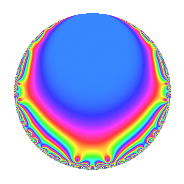Properties

 Label 4010.2.a.gLevel 4010 Weight 2 Character orbit 4010.a Self dual Yes Analytic conductor 32.020 Analytic rank 1 Dimension 2 CM No Inner twists 1

Related objects

Newspace parameters

 Level: $$N$$ = $$4010 = 2 \cdot 5 \cdot 401$$ Weight: $$k$$ = $$2$$ Character orbit: $$[\chi]$$ = 4010.a (trivial)

Newform invariants

 Self dual: Yes Analytic conductor: $$32.0200112105$$ Analytic rank: $$1$$ Dimension: $$2$$ Coefficient field: $$\Q(\sqrt{2})$$ Coefficient ring: $$\Z[a_1, a_2, a_3]$$ Coefficient ring index: $$1$$ Fricke sign: $$1$$ Sato-Tate group: $\mathrm{SU}(2)$

$q$-expansion

Coefficients of the $$q$$-expansion are expressed in terms of $$\beta = \sqrt{2}$$. We also show the integral $$q$$-expansion of the trace form.

 $$f(q)$$ $$=$$ $$q$$ $$- q^{2}$$ $$+ \beta q^{3}$$ $$+ q^{4}$$ $$+ q^{5}$$ $$- \beta q^{6}$$ $$+ 2 \beta q^{7}$$ $$- q^{8}$$ $$- q^{9}$$ $$+O(q^{10})$$ $$q$$ $$- q^{2}$$ $$+ \beta q^{3}$$ $$+ q^{4}$$ $$+ q^{5}$$ $$- \beta q^{6}$$ $$+ 2 \beta q^{7}$$ $$- q^{8}$$ $$- q^{9}$$ $$- q^{10}$$ $$-2 q^{11}$$ $$+ \beta q^{12}$$ $$+ ( -2 - \beta ) q^{13}$$ $$-2 \beta q^{14}$$ $$+ \beta q^{15}$$ $$+ q^{16}$$ $$- \beta q^{17}$$ $$+ q^{18}$$ $$-2 q^{19}$$ $$+ q^{20}$$ $$+ 4 q^{21}$$ $$+ 2 q^{22}$$ $$+ ( 2 - \beta ) q^{23}$$ $$- \beta q^{24}$$ $$+ q^{25}$$ $$+ ( 2 + \beta ) q^{26}$$ $$-4 \beta q^{27}$$ $$+ 2 \beta q^{28}$$ $$-6 \beta q^{29}$$ $$- \beta q^{30}$$ $$-4 q^{31}$$ $$- q^{32}$$ $$-2 \beta q^{33}$$ $$+ \beta q^{34}$$ $$+ 2 \beta q^{35}$$ $$- q^{36}$$ $$+ ( 6 - 3 \beta ) q^{37}$$ $$+ 2 q^{38}$$ $$+ ( -2 - 2 \beta ) q^{39}$$ $$- q^{40}$$ $$+ 4 \beta q^{41}$$ $$-4 q^{42}$$ $$-8 \beta q^{43}$$ $$-2 q^{44}$$ $$- q^{45}$$ $$+ ( -2 + \beta ) q^{46}$$ $$+ ( -4 - 2 \beta ) q^{47}$$ $$+ \beta q^{48}$$ $$+ q^{49}$$ $$- q^{50}$$ $$-2 q^{51}$$ $$+ ( -2 - \beta ) q^{52}$$ $$+ ( 2 + 3 \beta ) q^{53}$$ $$+ 4 \beta q^{54}$$ $$-2 q^{55}$$ $$-2 \beta q^{56}$$ $$-2 \beta q^{57}$$ $$+ 6 \beta q^{58}$$ $$+ ( 6 + 4 \beta ) q^{59}$$ $$+ \beta q^{60}$$ $$+ 8 q^{61}$$ $$+ 4 q^{62}$$ $$-2 \beta q^{63}$$ $$+ q^{64}$$ $$+ ( -2 - \beta ) q^{65}$$ $$+ 2 \beta q^{66}$$ $$+ ( -4 - 5 \beta ) q^{67}$$ $$- \beta q^{68}$$ $$+ ( -2 + 2 \beta ) q^{69}$$ $$-2 \beta q^{70}$$ $$+ ( -8 + 2 \beta ) q^{71}$$ $$+ q^{72}$$ $$+ ( -2 - 6 \beta ) q^{73}$$ $$+ ( -6 + 3 \beta ) q^{74}$$ $$+ \beta q^{75}$$ $$-2 q^{76}$$ $$-4 \beta q^{77}$$ $$+ ( 2 + 2 \beta ) q^{78}$$ $$+ ( -12 + 4 \beta ) q^{79}$$ $$+ q^{80}$$ $$-5 q^{81}$$ $$-4 \beta q^{82}$$ $$+ ( -4 + 6 \beta ) q^{83}$$ $$+ 4 q^{84}$$ $$- \beta q^{85}$$ $$+ 8 \beta q^{86}$$ $$-12 q^{87}$$ $$+ 2 q^{88}$$ $$-10 q^{89}$$ $$+ q^{90}$$ $$+ ( -4 - 4 \beta ) q^{91}$$ $$+ ( 2 - \beta ) q^{92}$$ $$-4 \beta q^{93}$$ $$+ ( 4 + 2 \beta ) q^{94}$$ $$-2 q^{95}$$ $$- \beta q^{96}$$ $$+ ( -12 - \beta ) q^{97}$$ $$- q^{98}$$ $$+ 2 q^{99}$$ $$+O(q^{100})$$ $$\operatorname{Tr}(f)(q)$$ $$=$$ $$2q$$ $$\mathstrut -\mathstrut 2q^{2}$$ $$\mathstrut +\mathstrut 2q^{4}$$ $$\mathstrut +\mathstrut 2q^{5}$$ $$\mathstrut -\mathstrut 2q^{8}$$ $$\mathstrut -\mathstrut 2q^{9}$$ $$\mathstrut +\mathstrut O(q^{10})$$ $$2q$$ $$\mathstrut -\mathstrut 2q^{2}$$ $$\mathstrut +\mathstrut 2q^{4}$$ $$\mathstrut +\mathstrut 2q^{5}$$ $$\mathstrut -\mathstrut 2q^{8}$$ $$\mathstrut -\mathstrut 2q^{9}$$ $$\mathstrut -\mathstrut 2q^{10}$$ $$\mathstrut -\mathstrut 4q^{11}$$ $$\mathstrut -\mathstrut 4q^{13}$$ $$\mathstrut +\mathstrut 2q^{16}$$ $$\mathstrut +\mathstrut 2q^{18}$$ $$\mathstrut -\mathstrut 4q^{19}$$ $$\mathstrut +\mathstrut 2q^{20}$$ $$\mathstrut +\mathstrut 8q^{21}$$ $$\mathstrut +\mathstrut 4q^{22}$$ $$\mathstrut +\mathstrut 4q^{23}$$ $$\mathstrut +\mathstrut 2q^{25}$$ $$\mathstrut +\mathstrut 4q^{26}$$ $$\mathstrut -\mathstrut 8q^{31}$$ $$\mathstrut -\mathstrut 2q^{32}$$ $$\mathstrut -\mathstrut 2q^{36}$$ $$\mathstrut +\mathstrut 12q^{37}$$ $$\mathstrut +\mathstrut 4q^{38}$$ $$\mathstrut -\mathstrut 4q^{39}$$ $$\mathstrut -\mathstrut 2q^{40}$$ $$\mathstrut -\mathstrut 8q^{42}$$ $$\mathstrut -\mathstrut 4q^{44}$$ $$\mathstrut -\mathstrut 2q^{45}$$ $$\mathstrut -\mathstrut 4q^{46}$$ $$\mathstrut -\mathstrut 8q^{47}$$ $$\mathstrut +\mathstrut 2q^{49}$$ $$\mathstrut -\mathstrut 2q^{50}$$ $$\mathstrut -\mathstrut 4q^{51}$$ $$\mathstrut -\mathstrut 4q^{52}$$ $$\mathstrut +\mathstrut 4q^{53}$$ $$\mathstrut -\mathstrut 4q^{55}$$ $$\mathstrut +\mathstrut 12q^{59}$$ $$\mathstrut +\mathstrut 16q^{61}$$ $$\mathstrut +\mathstrut 8q^{62}$$ $$\mathstrut +\mathstrut 2q^{64}$$ $$\mathstrut -\mathstrut 4q^{65}$$ $$\mathstrut -\mathstrut 8q^{67}$$ $$\mathstrut -\mathstrut 4q^{69}$$ $$\mathstrut -\mathstrut 16q^{71}$$ $$\mathstrut +\mathstrut 2q^{72}$$ $$\mathstrut -\mathstrut 4q^{73}$$ $$\mathstrut -\mathstrut 12q^{74}$$ $$\mathstrut -\mathstrut 4q^{76}$$ $$\mathstrut +\mathstrut 4q^{78}$$ $$\mathstrut -\mathstrut 24q^{79}$$ $$\mathstrut +\mathstrut 2q^{80}$$ $$\mathstrut -\mathstrut 10q^{81}$$ $$\mathstrut -\mathstrut 8q^{83}$$ $$\mathstrut +\mathstrut 8q^{84}$$ $$\mathstrut -\mathstrut 24q^{87}$$ $$\mathstrut +\mathstrut 4q^{88}$$ $$\mathstrut -\mathstrut 20q^{89}$$ $$\mathstrut +\mathstrut 2q^{90}$$ $$\mathstrut -\mathstrut 8q^{91}$$ $$\mathstrut +\mathstrut 4q^{92}$$ $$\mathstrut +\mathstrut 8q^{94}$$ $$\mathstrut -\mathstrut 4q^{95}$$ $$\mathstrut -\mathstrut 24q^{97}$$ $$\mathstrut -\mathstrut 2q^{98}$$ $$\mathstrut +\mathstrut 4q^{99}$$ $$\mathstrut +\mathstrut O(q^{100})$$

Embeddings

For each embedding $$\iota_m$$ of the coefficient field, the values $$\iota_m(a_n)$$ are shown below.

For more information on an embedded modular form you can click on its label.

Label $$\iota_m(\nu)$$ $$a_{2}$$ $$a_{3}$$ $$a_{4}$$ $$a_{5}$$ $$a_{6}$$ $$a_{7}$$ $$a_{8}$$ $$a_{9}$$ $$a_{10}$$
1.1
 −1.41421 1.41421
−1.00000 −1.41421 1.00000 1.00000 1.41421 −2.82843 −1.00000 −1.00000 −1.00000
1.2 −1.00000 1.41421 1.00000 1.00000 −1.41421 2.82843 −1.00000 −1.00000 −1.00000
 $$n$$: e.g. 2-40 or 990-1000 Significant digits: Format: Complex embeddings Normalized embeddings Satake parameters Satake angles

Inner twists

This newform does not admit any (nontrivial) inner twists.

Atkin-Lehner signs

$$p$$ Sign
$$2$$ $$1$$
$$5$$ $$-1$$
$$401$$ $$-1$$

Hecke kernels

This newform can be constructed as the intersection of the kernels of the following linear operators acting on $$S_{2}^{\mathrm{new}}(\Gamma_0(4010))$$:

 $$T_{3}^{2}$$ $$\mathstrut -\mathstrut 2$$ $$T_{7}^{2}$$ $$\mathstrut -\mathstrut 8$$ $$T_{11}$$ $$\mathstrut +\mathstrut 2$$